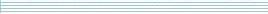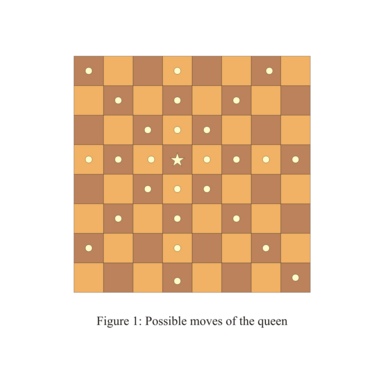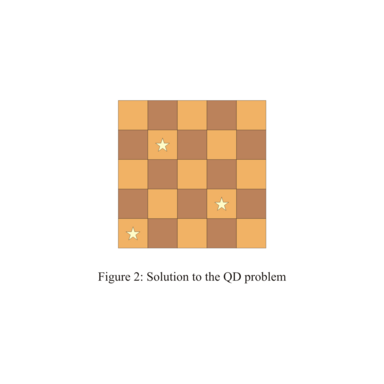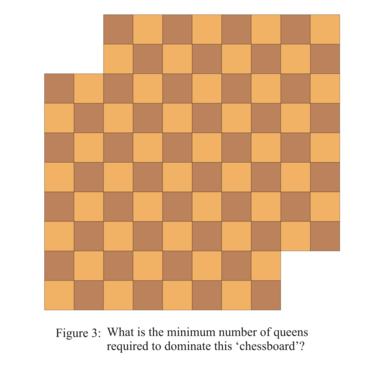| home      | people     | research     | publications    | seminars    | events     | contactCommunication Networks   | Systems Biology   | Hybrid Systems    | Machine Learning   | Dynamics & Interaction### Schools Mathematics Grand Challenge

PROBLEM 19:

One of the most famous sequences of numbers in mathematics is the "Fibonacci sequence". The rules that describe this famous sequence of numbers are the following:

1. The first number in the sequence is 1;
2. The second number in the sequence is 1;
3. Every number from the third number on is the sum of the previous two numbers - so the third number is 1 + 1 = 2, the fourth number is 2 + 1 = 3 and so on.

So, the first few numbers in the Fibonacci sequence are:

1, 1, 2, 3, 5, 8, 13, 21

What is the units digit of the 1200th number in the Fibonacci sequence?

PROBLEM 20:

The queen domination problem (QDP for short) is a problem in mathematics inspired by chess. On a normal chessboard with 64 squares the QDP may be phrased as follows: given a certain number of queens, place them on the board in such a way that each of the 64 squares is either covered by a queen or under attack by a queen.

We say that a square is under attack (by a queen) if it is contained in the set of possible queen moves. Recall that a queen can move any number of squares in a straight line vertically, horizontally, or diagonally, as illustrated below. Thus, in this example, the squares under attack are the squares marked with solid yellow circles. The star-shaped symbol respresent a square occupied by a queen.Going back to our queen domination problem now. The picture below shows a solution to a QDP for a chessboard with 5x5 squares. For this case, the number of queens, 3, is the smallest number of queens that yields a solution to the QDP. In other words: there is no way of 'dominating' a 5x5 chessboard with 2 queens (or 1 for that matter).Now consider the`chessboard' below and answer the following question: what is the minimum number of queens needed to occupy or attack all 92 squares on this modified chessboard.HINT: the miminum number of queens needed to solve the QDP for a regular 8x8 chessboard is 5.

PROBLEM 21:

If n is a whole number, then

M = 440 + 41024 + 4n

is also a whole number. However, M isn't always the square of a whole number. What is the the biggest n so that M is the square of a whole number?

NOTE: In this question, 440 means 4 multiplied by itself 40 times, 41024 means 4 multiplied by itself 1024 times and 4n means 4 multiplied by itself n times.

HINT: if no one finds the biggest possible n, we will give credit for the largest submitted n that makes M the square of a whole number.